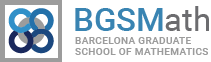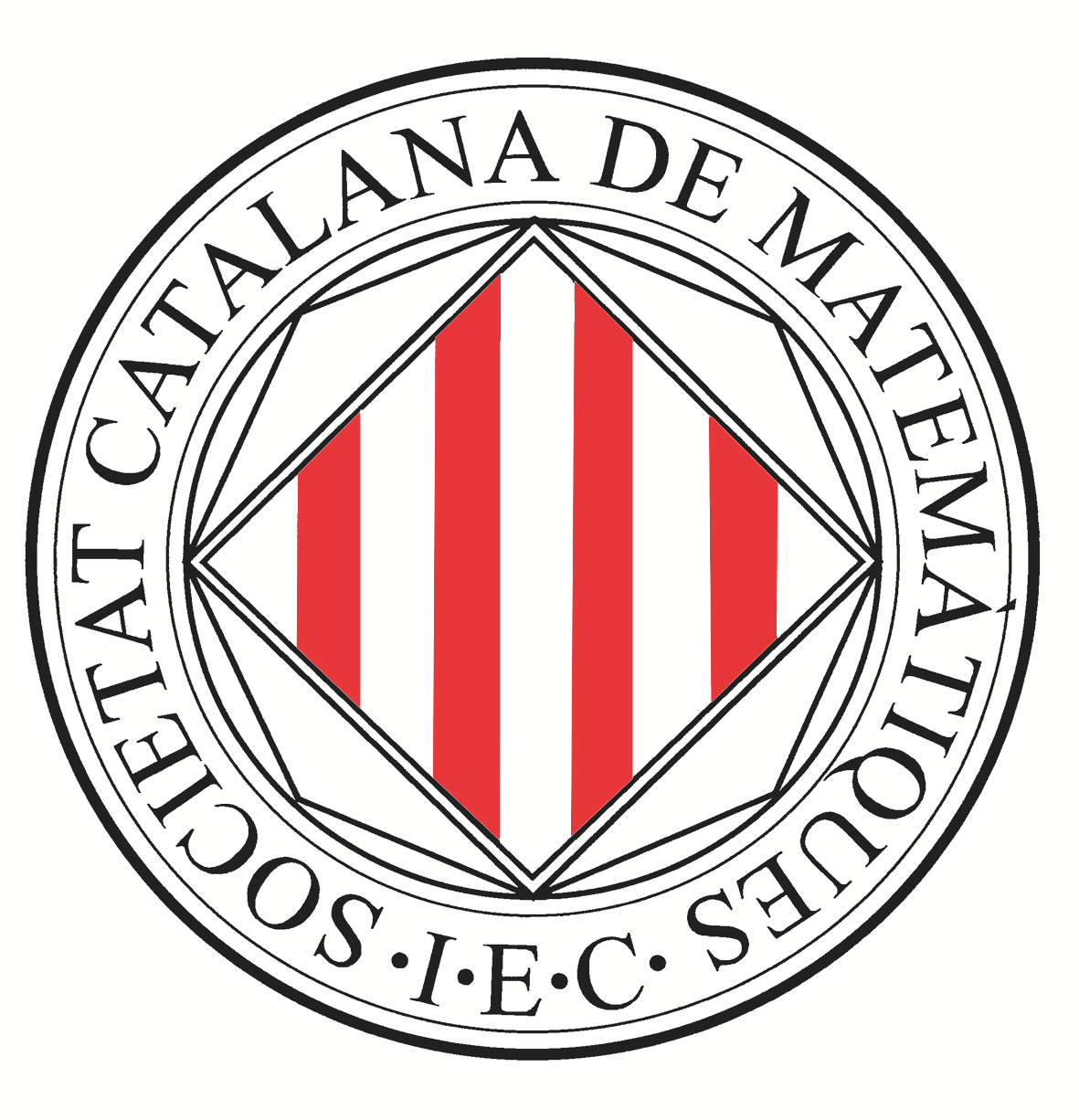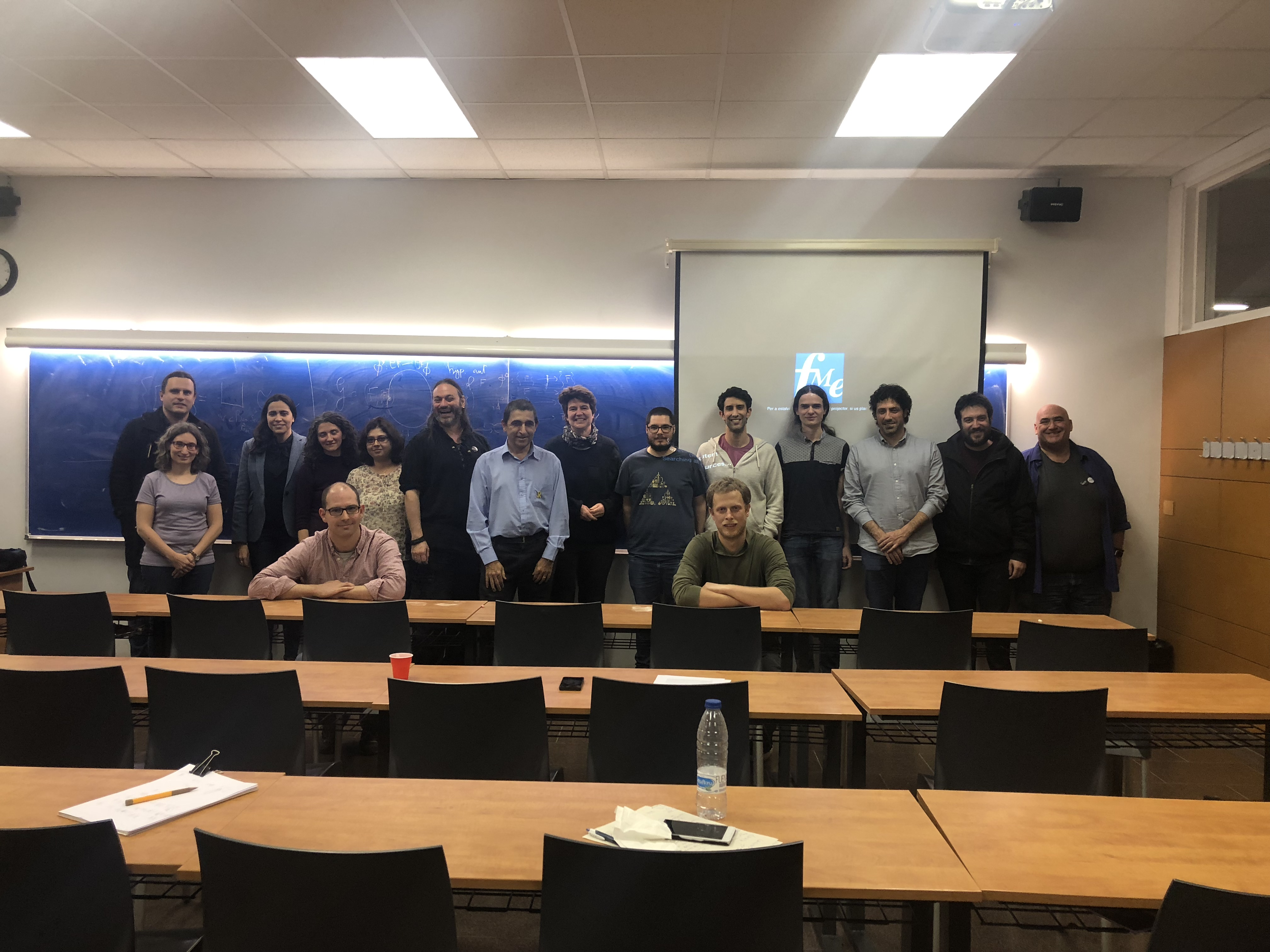# Fourteenth Barcelona Weekend in Group Theory

## March 15th and 16th, 2019

The Fourteenth Barcelona Weekend in Group Theory will take place on March 15th and 16th, 2019 at the Facultat de Matemàtiques i Estadística and the Escola Politècnica Superior d'Enginyeria de Manresa of the Universitat Politècnica de Catalunya.

Friday March 15th, 2019, Facultat de Matemàtiques i Estadística, Universitat Politècnica de Catalunya, carrer Pau Gargallo 14, Barcelona.

• 15:00-16:00 Brita Nucinkis (Royal Holloway, University of London, England). An irrational slope Thompson's group

• In this talk I will discuss a relative to Thompson's group $F$, the group $F_\tau,$ which is the group of piecewise linear homeomorphisms of $[0,1]$ with breakpoints in $\mathbb{Z}[\tau]$ and slopes powers of $\tau,$ where $\tau = \frac{\sqrt5 -1}{2}$ is the small Golden Ratio. This group was first considered by S. Cleary, who showed that the group was finitely presented and of type $\operatorname{F}_\infty.$ Here we take a combinatorial approach considering elements as tree-pair diagrams, where the trees are finite binary trees, but with two different kinds of carets. We use this representation to show that the commutator subgroup is simple, give a unique normal form, and study some metric properties of this group. This is joint work with J. Burillo and L. Reeves.

• 16:15-17:15 Dionysis Syrigos (University of Southampton, England). Min-Set of an Irreducible automorphism of a free product

• Let G be a group which splits as G = F_n * G_1 *...*G_k, where every G_i is freely indecomposable and not isomorphic to the group of integers. For Guirardel and Levitt generalised the Culler- Vogtmann Outer space of a free group by introducing an Outer space on which Out(G) acts. Francaviglia and Martino introduced the Lipschitz metric for the Culler- Vogtmann space and later for the general Outer space. For any automorphism of Out(G), we can define the displacement function with respect to the Lipschitz metric and the corresponding level sets. Recently, the same authors proved that for every L, the L-level set (the set of points of the outer space which are displaced by at most L) is connected, whenever it is non-empty . In the special case of an irreducible automorphism, they proved that the Min- set (the set of points which are minimally displaced) is always non-empty and it coincides with the set of the points that admit train track representatives for the automorphism. In a joint paper with Francaviglia and Martino, we prove that the Min set of an irreducible automorphism (with exponential growth), is locally finite, even if the relative outer space is locally infinite.

• 17:30-18:30 Federico Berlai (Euskal Herriko Uniberstsitatea/Universidad del País Vasco, Bilbao, Spain). A combination theorem for hierarchically hyperbolic groups

• Hierarchically hyperbolic groups (HHGs) and spaces are recently-introduced generalisations of (Gromov-)hyperbolic groups and spaces. Other examples of HHGs include mapping class groups, right-angled Artin groups, and many groups acting properly and cocompactly on CAT(0) cube complexes. In this talk, after introducing and motivating them, I will present a combination theorem for hierarchically hyperbolic groups. As a corollary, any graph product of finitely many HHGs is itself a HHG. Joint work with B. Robbio.

• 18:45-19:45 Motiejus Valiunas (University of Southampton, England). Probabilistic nilpotency in infinite groups

Saturday March 16th, 2019, Escola Politècnica Superior d'Enginyeria de Manresa, Av. de les Bases de Manresa, 61-73, Manresa.
• 10:30-11:30 Natàlia Castellana (Universidad Autònoma de Barcelona, Spain). Stratification of the module category for homotopical groups

• Group cohomology is a subject in which both algebra an topology interact in a bidirectional way. One of the most relevant results is that of Quillen stratification theorem on the spectrum of the cohomology ring which is described in terms of the information provided by elementary abelian p-subgrpups. Consider the category of modules over $kG$ where $G$ is finite, $ch(k)=p$ with $p| |G|$, Benson-Iyengar-Krause classified the localising subcategories by means of subsets of the spectrum of the cohomology and where Quillen's stratification result is a key ingredient. With an appropiate version of the module category, stratification results extend to compact Lie groups (Benson-Grenlees) and classifying spaces of fusion systems (algebraic structures which provide homotopical analogues for $p$-completions of classifying spaces of finite groups).

• 11:45-12:45 Ramón Flores (Universidad de Sevilla, Spain). Growth of braid monoids and the partial theta function.

• We will show how to compute the growth function of a braid monoid with respect to the standard generators as the inverse of the determinant of a matrix that can be easily described. In this way, it is possible to obtain the limit of the sequence of growth rates of the monoids A_n, which turns to be the growth rate of the unique solution of a classical partial theta function. Moreover, we prove that the coefficients of this solution can be obtained just counting braids. This is an unexpected new connection between Braid Theory and Analysis. Joint work with Juan González-Meneses.

There is no registration, but if you are interested in attending, please let us know at our email addresses enric.ventura@upc.edu or pep.burillo@upc.edu. We need to know approximately how many people will be coming.
This event is sponsored by:
Barcelona Graduate School in Mathematics:Societat Catalana de Matemàtiques:After the talks on Saturday, we will have a traditional Catalan Calçotada, with Spring onions with special sauce and barbecue.